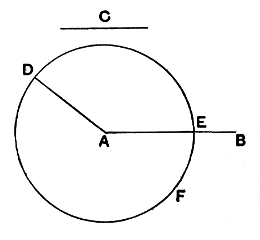#Elements: Book I, Proposition 3

Math Lair Home > Source Material > Elements > Book I, Proposition 3

The following is as given in Sir Thomas L. Heath's translation, which can be found in the book The Thirteen Books of The Elements, Vol. 1.

Elements on the Math Lair
Book IBook IIBook IX
Definitions, Postulates, and Common NotionsDefinitionsProposition 20
Proposition 1, Proposition 3, Proposition 14Proposition 36
Proposition 5, Proposition 6,
Proposition 29, Proposition 47

## Proposition 3.

Given two unequal straight lines, to cut off from the greater a straight line equal to the less.Let AB, C be the two given unequal straight lines, and let AB be the greater of them.

Thus it is required to cut off from AB the greater a straight line equal to C the less.

At the point A let AD be placed equal to the straight line C; [I. 2]
and with centre A and distance AD let the circle DEF be described. [Post. 3]

Now, since the point A is the centre of the circle DEF,

AE is equal to AD. [Def. 15]

But C is also equal to AD.

Therefore ach of the straight lines AE, C is equal to AD; so that AE is also equal to C. [C. N. 1]

Therefore, given the two straight lines AB, C, from AB the greater AE has been cut off equal to C the less.

(Being) what it was required to do.